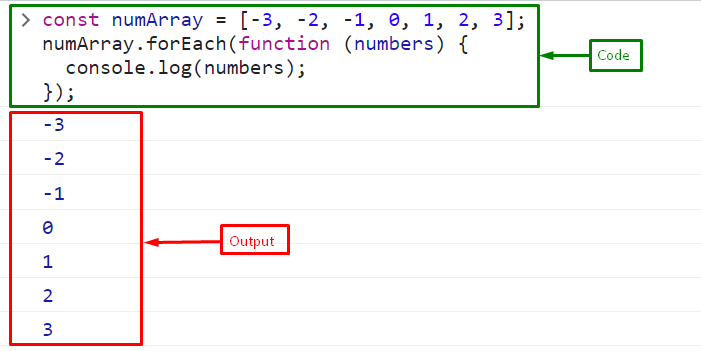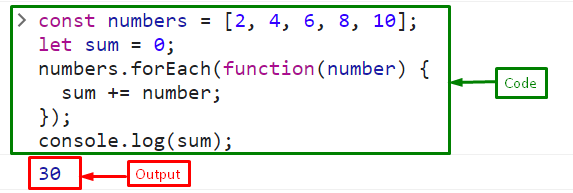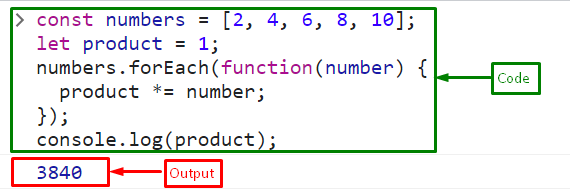# What is “forEach()” Method in JavaScript? (With Examples)

In JavaScript, the term “for each” is not a specific construct or method. However, JavaScript provides several iteration methods that can be used to iterate over elements in an array or values in an object. One such example of an iteration method is the “forEach()” method. It iterates/loops through every element of an array and performs a specified action for each element. It gives a quick and easy way to loop through an array without using a standard “for” loop.

This tutorial will describe the for each in JavaScript.

## What is “forEach()” Method in JavaScript?

The “forEach()” method is an iterative method for looping through each element of an array and performing a particular action on them. It uses a callback function as an argument that is executed for each array element. The forEach() method does not create a new array; instead, it iterates across the existing array.

It is particularly useful when the same operation or action performs on each element of an array. It provides a clean and concise syntax, reducing the need for manual indexing and looping.

Syntax

The given syntax is used for the “forEach()” method in JavaScript:

`forEach(callbackFn)`

Example 1

First, create an array named “numArray”:

`const numArray = [-3, -2, -1, 0, 1, 2, 3];`

Call the “forEach()” method to iterate the array elements and print them on the console:

```numArray.forEach(function (numbers) {
console.log(numbers);
});```

The output indicates that the “forEach()” method has been successfully iterated over the array and displayed on the console:Example 2

In the given example, we will iterate the array and print the sum of all elements using the forEach() method:

`const numbers = [2, 4, 6, 8, 10];`

Initialize a variable “sum” with value “0”:

`let sum = 0;`

Call the forEach() method that will iterate the array and outputs the sum of all the array elements:

```numbers.forEach(function(number) {
sum += number;
});```

Finally, display the sum on the console with the help of the “console.log()” method:

`console.log(sum);`

OutputExample 3

Here, we will multiply all the array elements and print the result on the console:

`let product = 1;`

Call the forEach() method on the array to iterate every element and multiply them:

```numbers.forEach(function(number) {
product *= number;});```

Lastly, display the product on the console:

`console.log(product);`

OutputThat’s all about the for each in JavaScript.

## Conclusion

for each” is not a specific construct or method however, JavaScript provides several iteration methods such as the “forEach()” method that can be used to iterate over elements in an array or values in an object. It uses a callback function which is executed for every array element. This tutorial described for each in JavaScript.